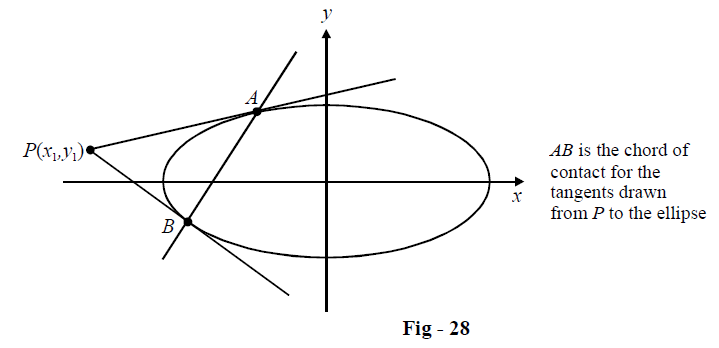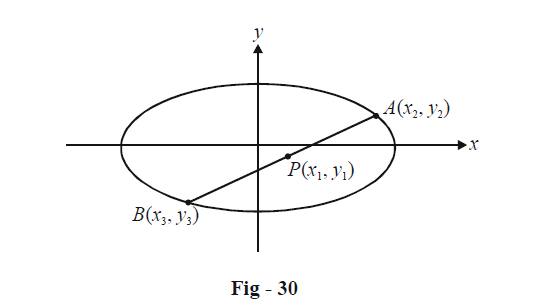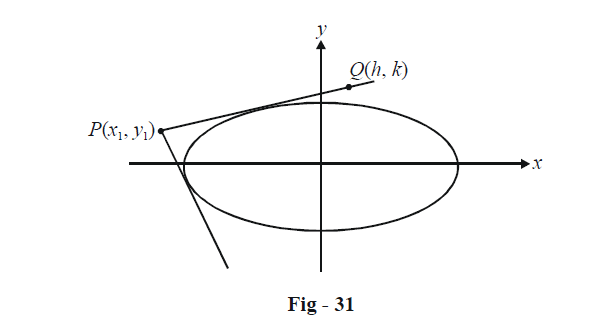# Important Results On Tangents And Chords Of Ellipses

Go back to  'Ellipse'

We will find, in this section, that the equations of chord of contact, chord bisected at a given point, and pair of tangents from a point take the same form as they do in the case of circles and parabolas. We will use the ellipse $$S(x,\,y) \equiv \frac{{{x^2}}}{{{a^2}}} + \frac{{{y^2}}}{{{b^2}}} - 1 = 0$$ in this discussion.

CHORD OF CONTACT FROM P(x1, y1): Two tangents are drawn from an external point P(x1, y1) to the ellipse  $$\frac{{{x^2}}}{{{a^2}}} + \frac{{{y^2}}}{{{b^2}}} = 1,$$ touching this ellipse at points A and B. We need to find the equation of AB, the chord of contact.To determine this equation, we’ll follow precisely the same approach as we did in the case of circles and parabolas.

Assume the coordinates of A and B to be (x2, y2) and (x3, y3) respectively. The tangents at A and B then have the equations :

$\frac{{x{x_2}}}{{{a^2}}} + \frac{{y{y_2}}}{{{b^2}}} = 1\;\text{and}\;\frac{{x{x_3}}}{{{a^2}}} + \frac{{y{y_3}}}{{{b^2}}} = 1$

Since these tangents intersect at $$P,({x_1},{y_1})$$ must satisfy both these equations. Thus,

$\frac{{{x_1}{x_2}}}{{{a^2}}} + \frac{{{y_1}{y_2}}}{{{b^2}}} = 1\;\text{and}\;\frac{{{x_1}{x_3}}}{{{a^2}}} + \frac{{{y_1}{y_3}}}{{{b^2}}} = 1$

Now, from these two equations, we can deduce that $$({x_2},{y_2})\;\text{and}\;({x_3},{y_3})$$ and are actually two solutions of the linear equation

$\frac{{x{x_1}}}{{{a^2}}} + \frac{{y{y_1}}}{{{b^2}}} = 1$

which means that this must be the chord of contact.

The equation of the chord of contact can be written concisely as

$\boxed{T({x_1},{y_1}) = 0}$

CHORD BISECTED AT P(x1, y1):Let the required chord be AB, where the coordinates of A and B are (x2, y2) and (x3, y3) respectively.Since A and B lie on the ellipse, we have

\begin{align}&\frac{{x_2^2}}{{{a^2}}} + \frac{{y_2^2}}{{{b^2}}} = 1\quad\quad\quad...\left( 1 \right)\\&\frac{{x_3^2}}{{{a^2}}} + \frac{{y_3^2}}{{{b^2}}} = 1\quad\quad\quad...\left( 2 \right)\end{align}

By (1) – (2), we have

$\frac{{({x_2} - {x_3})({x_2} + {x_3})}}{{{a^2}}} + \frac{{({y_2} + {y_3})({y_2} - {y_3})}}{{{b^2}}} = 0\quad\quad\quad...\left( 3 \right)$

We also have to use the fact that P is the mid-point of AB, so that

${x_2} + {x_3} = 2{x_1}and{y_2} + {y_3} = 2{y_1}\quad\quad\quad...\left( 4 \right)$

Using (4) in (3), we obtain

\begin{align}&\frac{{2{x_1}({x_2} - {x_3})}}{{{a^2}}} + \frac{{2{y_1}({y_2} - {y_3})}}{{{b^2}}} = 0\\& \Rightarrow \quad \frac{{{y_2} - {y_3}}}{{{x_2} - {x_3}}} = - \frac{{{b^2}{x_1}}}{{{a^2}{y_1}}}\quad\quad\quad...\left( 5 \right)\end{align}

Observe carefully that what (5) gives is simply the slope of AB.

Once we know the slope of AB, we can use the point-slope form to write its equation as :

\begin{align}&y - {y_1} = - \frac{{{b^2}{x_1}}}{{{a^2}{y_1}}}(x - {x_1})\\ &\Rightarrow \quad \frac{{x{x_1}}}{{{a^2}}} + \frac{{y{y_1}}}{{{b^2}}} = \frac{{x_1^2}}{{{a^2}}} + \frac{{y_1^2}}{{{b^2}}}\end{align}

If we subtract 1 from both sides, we have

$\frac{{x{x_1}}}{{{a^2}}} + \frac{{y{y_1}}}{{{b^2}}} - 1 = \frac{{x_1^2}}{{{a^2}}} + \frac{{y_1^2}}{{{b^2}}} - 1$

which can be written concisely as

$\boxed{T({x_1},{y_1}) = S({x_1},{y_1})}$

PAIR OF TANGENTS FROM P(x1, y1): Let Q(h, k) be any point on the pair of tangents drawn from P to the ellipse.The equation of PQ, by two-point form is

\begin{align}&\frac{{y - {y_1}}}{{x - {x_1}}} = \frac{{k - {y_1}}}{{h - {x_1}}}\\& \Rightarrow \quad y = \left( {\frac{{k - {y_1}}}{{h - {x_1}}}} \right)x + \left( {\frac{{h{y_1} - k{x_1}}}{{h - {x_1}}}} \right)\quad\quad\quad...\left( 1 \right)\end{align}

PQ being a tangent to the ellipse, the condition $${c^2} = {a^2}{m^2} + {b^2}$$ for tangency must be satisfied in (1). Thus,

${\left( {\frac{{h{y_1} - k{x_1}}}{{h - {x_1}}}} \right)^2} = {a^2}{\left( {\frac{{k - {y_1}}}{{h - {x_1}}}} \right)^2} + {b^2}\quad\quad\quad...\left( 2 \right)$

As expected, (2) is a second degree equation in h and k, since the point Q(h, k) can lie on essentially  two different lines (the two tangents). Thus, what (2) represents is the pair of tangents from P to the ellipse.

After some manipulation, the relation in (2) can be written as

$\left( {\frac{{{h^2}}}{{{a^2}}} + \frac{{{k^2}}}{{{b^2}}} - 1} \right)\left( {\frac{{x_1^2}}{{{a^2}}} + \frac{{y_1^2}}{{{b^2}}} - 1} \right) = {\left( {\frac{{h{x_1}}}{{{a^2}}} + \frac{{k{y_1}}}{{{b^2}}} - 1} \right)^2}$

Using (x, y) instead of (h, k), the equation to the pair of tangents becomes

$\left( {\frac{{{x^2}}}{{{a^2}}} + \frac{{{y^2}}}{{{b^2}}} - 1} \right)\left( {\frac{{x_1^2}}{{{a^2}}} + \frac{{y_1^2}}{{{b^2}}} - 1} \right) = {\left( {\frac{{x{x_1}}}{{{a^2}}} + \frac{{y{y_1}}}{{{b^2}}} - 1} \right)^2}$

which can be written concisely as

$\boxed{T{{({x_1},{y_2})}^2} = S(x,y)S({x_1},{y_1})}$

or simply $${T^2} = S{S_1}.$$